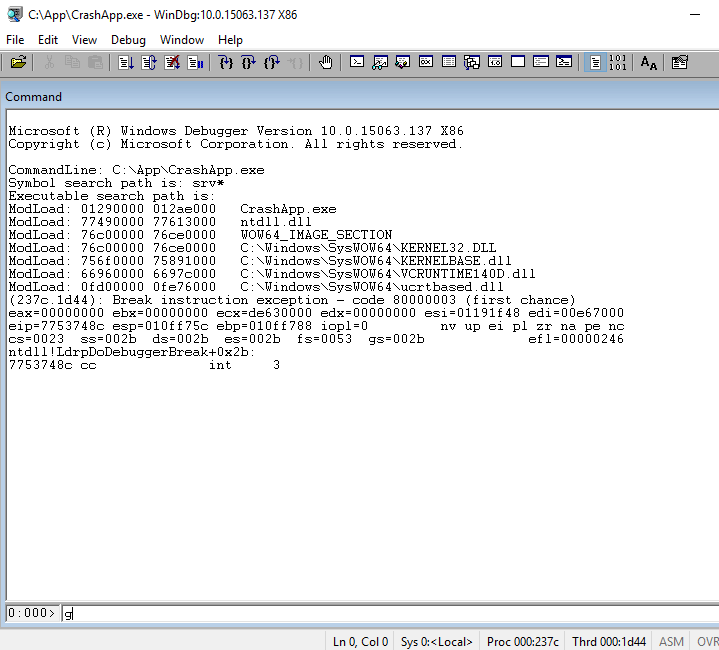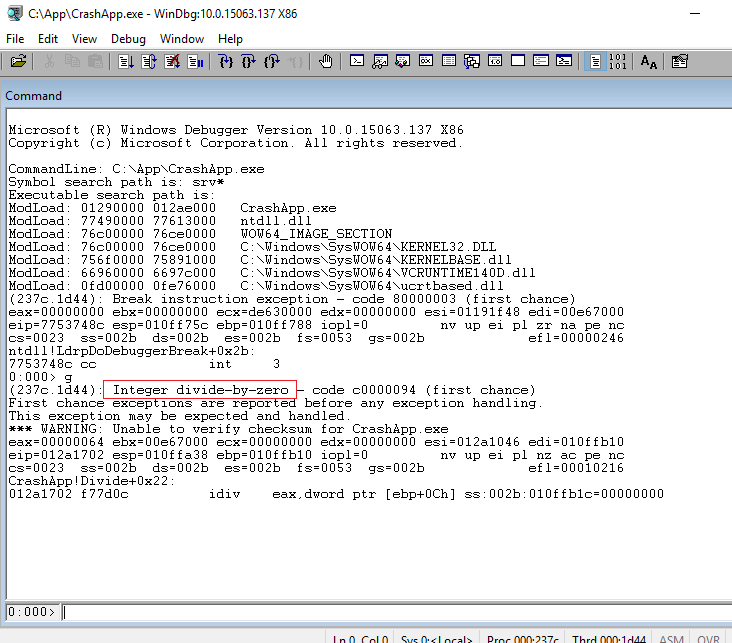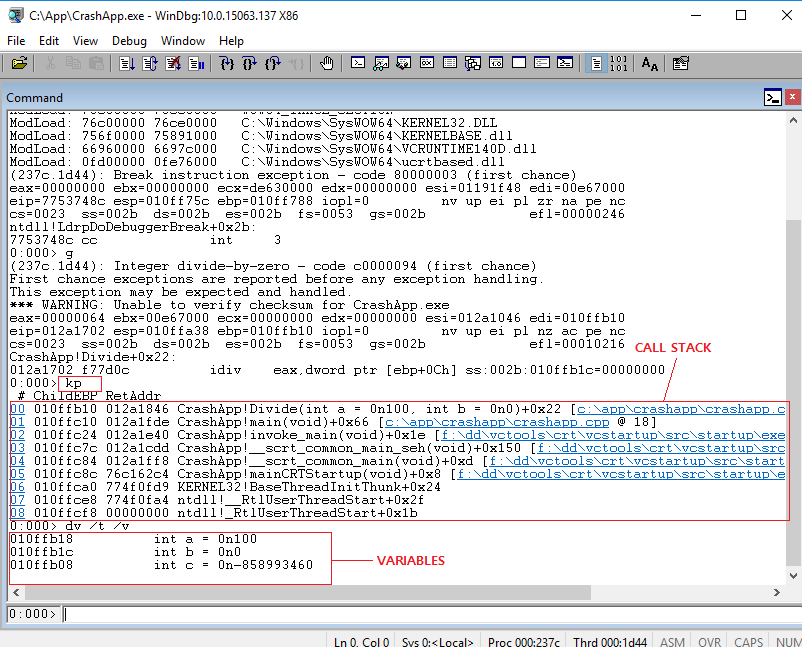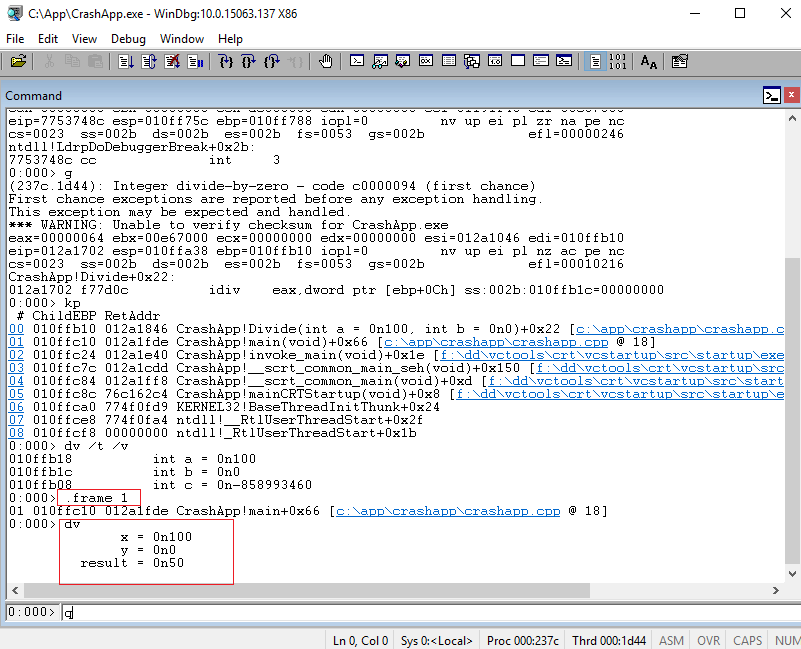WinDbg Basic Commands

#### WinDbg Basic Commands

When WinDbg is attached to the debuggee process, WinDbg can fully control any execution of the debuggee process. For example, WinDbg can run the debuggee step by step, check call stack of a thread, check variable/data structure values, and set breakpoint on a specific code, and so on.

Let's look at some of typical commands that are used in WinDbg. First, here is a very simple C++ code we are going to use for debugging.

```#include "stdafx.h"

int Divide(int a, int b)
{
int c = a / b;
return c;
}

int main()
{
int x = 100;
int y = 2;

int result = Divide(x, y);
printf("%d\n", result);

y -= 2;
result = Divide(x, y);
printf("%d\n", result);

return 0;
}
```

After build, open executable file (call it CrashApp.exe) from WinDbg. When WinDbg opens EXE file, it immediately breaks into the beginning of the executable, which gives WinDbg an oppurtunity of any necessary control. If we want to set breakpoint or any other settings, this is the good time to do that. Once those settings are done (if any), we can issue g (go) command to keep running the debuggee.After g command, the debuggee application was crashed with divide by zero exception as shown below. If there is no exception, the debuggee will keep running.To check where the exception was thrown, we can use kp command which will show call stacks with input parameters information. Call stack shows all the function call history up to this exception. Each line in call stack is called "frame." Typically we tend to check call stack from top frame (latest call) to bottom frame. Each frame represent different function, so each frame has different variables. To check variables in current frame, use dv (display variables) command. If /t is used, variable type is also displayed and if /v is used, memory address is also displayed. As shown in the picture, we got divide by zero exception because variable b is 0.In order to change call stack frame, use .frame {frame#} command. For example, to go to the first frame, use ".frame 0" and for the second frame, use ".frame 1" (Please note that there is dot(.) in front of the command, which will be covered later). In below picture, we go to 2nd frame and check variables of main() function. Here we can see y value is zero, which is passed to 2nd parameter of Divide() function.Once all the investigation is done, use q (quit) command to exit WinDbg.